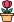엔지니어 게시판
LeetCode 솔루션 분류

# [11/25] 907. Sum of Subarray Minimums

### 작성자 정보

•작성
• 작성일

• 269 조회
• 1 댓글
• 0 추천
• 0 비추천

### 본문

Medium

Given an array of integers arr, find the sum of `min(b)`, where `b` ranges over every (contiguous) subarray of `arr`. Since the answer may be large, return the answer modulo `109 + 7`.

Example 1:

```Input: arr = [3,1,2,4]
Output: 17
Explanation:
Subarrays are , , , , [3,1], [1,2], [2,4], [3,1,2], [1,2,4], [3,1,2,4].
Minimums are 3, 1, 2, 4, 1, 1, 2, 1, 1, 1.
Sum is 17.
```

Example 2:

```Input: arr = [11,81,94,43,3]
Output: 444
```

Constraints:

• `1 <= arr.length <= 3 * 104`
• `1 <= arr[i] <= 3 * 104`
Accepted
105,123
Submissions
305,819
태그

댓글 1

## 학부유학생님의 댓글

• 익명
• 작성일
Runtime: 1247 ms, faster than 43.50% of Python3 online submissions for Sum of Subarray Minimums.
Memory Usage: 18.8 MB, less than 83.15% of Python3 online submissions for Sum of Subarray Minimums.
``````class Solution:
def sumSubarrayMins(self, arr: List[int]) -> int:
MOD = 10**9+7
res = 0
stack = []
arr =  + arr + 
for idx, num in enumerate(arr):
while stack and arr[stack[-1]] > num:
curr = stack.pop()
res += arr[curr]*(idx-curr)*(curr-stack[-1])
stack.append(idx)

return res%MOD``````
전체 349 / 1 페이지

• 등록일 10:47
• 등록일 07:18
• 등록일 02:04
• 등록일 02:03
• 등록일 00:48
• 등록일 02.01
• 등록일 02.01
• 등록일 02.01
• 등록일 02.01
• 등록일 02.01
• 등록일 02.01
• 등록일 02.01

• 등록일 01.31
• 등록일 01.31
• 등록일 01.31
• 등록일 01.27
• 등록일 01.27
• 등록일 01.23
• 등록일 01.23

### Stats

• 현재 접속자 74 명
• 오늘 방문자 1,352 명
• 어제 방문자 1,716 명
• 최대 방문자 2,853 명
• 전체 회원수 536 명